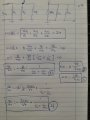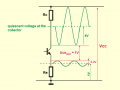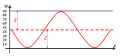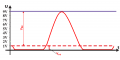# Help me design a common emitter amplifier.

#### babaliaris

Joined Nov 19, 2019
158
Since I'm tired now (being studying 2 days straight, seriously I didn't sleep) I will read your answers tomorrow, and see where my mistakes are.

#### babaliaris

Joined Nov 19, 2019
158
Ok, let's take it from scratch. I will stop being dependent on the internet and instead trust my own math and circuit theory knowledge.

Doing the following analysis (Without taking Rsig into consideration):Since $$\frac{1}{rce}$$ is pretty small, because $$rce = \frac{|A|}{I_{C_{dc}}}$$ and |A| pretty big like 100V,
I can assume that $$\frac{1}{rce} -> \infty$$ and equation 4 becomes:

$\frac{V_{o}}{V_{in}} = A_{v} \approx - \frac{I_{C_{dc}}}{V_{T}}R_{C}$

If we solve for $$I_{C_{dc}}$$:
$I_{C_{dc}} = -A_{v}\frac{V_{T}}{R_{C}}$

My amp must have $$Z_{out} = R_{C} = 2kΩ$$ so:
$I_{C_{dc}} = -A_{v}\frac{V_{T}}{R_{C}} = -(-10)\frac{25mV}{2kΩ} = 125μΑ = 0.125mA$

Is this right though? This current "feels" very small.

Even if I choose the Ic and Vc myself from the middle of the load line, then the voltage gain formula is useless to me, can't give me anything else.

Before we continue calculating the rest, let's talk about this first (Don't tell me anything about Re, R1, R2, Ve, let me decide first and talk about it).

Last edited:

#### Jony130

Joined Feb 17, 2009
5,439
Is this right though?
Yes, the results you got are correct.
This current "feels" very small.
This is normal for such a simple circuit. Because in this simple amplifier configuration you do not have too much degree of design freedom. Because Av = gm*Rc = Ic/Vt *Rc = (Ic * Rc)/Vt = V_rc/Vt. And if we set V_rc = Vcc/2 we get:
Av = Vcc/2/Vt = Vcc/(2Vt) = Vcc/50mV = 20*Vcc
And to provide ourselves with another degree of design freedom we add RE resistor into the mix. So we can "set the gain" independently.

•babaliaris

#### babaliaris

Joined Nov 19, 2019
158
I understand what you are saying. I will try to do it without Ce so that Re will be in the gain equation, but before that, I did the rest of the design and got a negative Resistance:

I'm reminding the specs of the amplifier:
Specs:
Zin = 10kΩ
Zout = 2kΩ
Av = -10
frequency response 20Hz - 20KHz
Vcc = 9V
$I_{C_{dc}} = -A_{v}\frac{V_{T}}{R_{C}} = -(-10)\frac{25mV}{2kΩ} = 125μΑ = 0.125mA$

$V_{R_{c}} = I_{C_{dc}}R_{C} = 0.125m \cdot 2k = 0.25V$

$V_{CC} - V_{C} = V_{R_{C}} <=> V_{C} = V_{CC} - V_{R_{C}} = 9 - 0.25 => V_{C} = 8.75V$

$V_{C} - V_{E} = \frac{V_{CC}}{2} = \frac{9}{2} = 4.5 <=>V_{E} = V_{C} - 4.5 = 8.75 - 4.5<=> V_{E} = 4.25V$

A note here: You and others are saying that we set Vc = Vcc/2. The graph though is Ic versus Vce, so this is
why I chose Vce = Vcc/2 instead. If this is a mistake, please explain to me why.

$V_{B} - V_{E} = 0.7 <=> V_{B} = V_{E} + 0.7 = 4.25 + 0.7 => V_{B} = 4.95V$

$R_{E} = \frac{I_{E} \approx I_{C_{dc}}}{V_{E}} = \frac{0.125m}{4.25} => R_{E} = 2.94 \cdot 10^{-5}Ω \approx 0$

So I decided that $$R_{E} = 0$$ and $$C_{E} = 0$$ (No point for a capacitor if there is no resistance at all).

Now I think the mistake is somewhere here and especially in what I'm about to do next:

$R_{2} = 10 \cdot re = 10\frac{V_{T}}{I_{C_{dc}}}\frac{B}{B+1} \approx 10\frac{V_{T}}{I_{C_{dc}}} = 10 \frac{25m}{0.125m} => R_{2} = 2kΩ$

$Z_{in} = R1//R2 = \frac{R_{1}R_{2}}{R1+R2} = 20k <=> R_{in} = \frac{R_{2}R_{in}}{R_{2}-R_{1}} = \frac{2k \cdot 20k}{2k - 10k} < 0$

Another mistake here I think is that Zin is actually: $$Z_{in} = Z_{1}//Z_{2}//rbe$$ where $rbe = H_{fe} \cdot \frac {V_{T}}{I_{C_{dc}}}$

Can you point out all my mistakes? Thank you!

Last edited:

#### Jony130

Joined Feb 17, 2009
5,439
Now I think the mistake is somewhere here and especially in what I'm about to do next:
RE = 4.25V/0.125mA = 34kΩ not 0Ω.

Also, notice one thing, because now V_rc = 0.25V thus Vc = 8.75V. You almost have no "space" for the output voltage to "swing up". Do you see it?
The voltage at the collector can swing from 8.75V down to Ve. The negative swing is 8.75V - 4.25V = 4.5V. But what about the positive swing?
Vc can go up only by 0.25V before it will hit Vcc rail.

•babaliaris

#### Jony130

Joined Feb 17, 2009
5,439
You and others are saying that we set Vc = Vcc/2. The graph though is Ic versus Vce, so this is
We are saying this because we need to have enough space to "fit" the output voltage swing.Vce = Vcc/2Vce = 1V#### Attachments

•babaliaris

#### babaliaris

Joined Nov 19, 2019
158
RE = 4.25V/0.125mA = 34kΩ not 0Ω.
Εmbarrassing calculations from my side. AnywayAlso, notice one thing, because now V_rc = 0.25V thus Vc = 8.75V. You almost have no "space" for the output voltage to "swing up". Do you see it?
The voltage at the collector can swing from 8.75V down to Ve. The negative swing is 8.75V - 4.25V = 4.5V. But what about the positive swing?
Vc can go up only by 0.25V before it will hit Vcc rail.
Yes, I totally get it. So this is why we want Vc = Vcc/2, so it can swing between Vcc | Vc | Ve the maximum way (and equally on both ways so Vpp = 2 times the peak of the output). Of course, Vc can't go below Ve because it will saturate the transistor. This actually raises a question:

Question 1:
Can Vin be big enough so at its negative T/2 (half period) will turn off the transistor by reducing Vb?

Question 2:
So what can I do here? Since I calculated Ic using the gain formula, and Rc=Zout, Vc is already determined to be 8.75V.
So, is the design problematic? Do I need to get rid of Ce and take Re into consideration in the gain formula? And why is the design problematic?

Thanks!

Last edited:

#### Jony130

Joined Feb 17, 2009
5,439
Question 1:
Can Vin be big enough so at its negative T/2 (half period) will turn off the transistor by reducing Vb?
Yes, it can. It is true for every amplifier in the world. If you apply too large an input signal to the amplifier input the amp will start to clip the signal (the signal will be distorted). Of course, this is undesired.

Question 2:
So what can I do here? Since I calculated Ic using the gain formula, and Rc=Zout, Vc is already determined to be 8.75V.
So, is the design problematic? Do I need to get rid of Ce and take Re into consideration in the gain formula? And why is the design problematic?
As I said earlier this is a very common problem when we are dealing with simple circuits. You demand too much from such a single transistor circuit.
We don't have almost any degree of freedom in choosing the component values. Because a single resistor has a big effect on multiple amplifier parameters at once.
This is why real-world amplifiers will have a much more complicated structure. In your case try to add RE resistor so you will get one degree of freedom. I hope that you understand what I meant.

Last edited:
•babaliaris

#### babaliaris

Joined Nov 19, 2019
158
Yes Totally! With the Re, I will have a little bit more freedom because it will allow me to use the gain formula for calculating Re AND will allow me to choose the two parameters (Ic, Vc) myself.

Also, I understand why with the capacitor Ce I had the Vc=8.75 reducing my amplification room. As you said, I asked for a gain of 10 which the transistor can probably give me, but in order for this to work, the math told me that in order for this gain to work you need Vc=8.75. But there is not a lot of room left above 8.75V, so even if I get the amplification my signal will be clipped.

So by solving one problem (Getting the gain I wanted), I'm introducing another, and no matter what I do, I can't achieve an equilibrium between those too unless I use more transistors. I will see in the future how with more transistors this will be solved, for now, let's try the Re alternative.

#### Audioguru again

Joined Oct 21, 2019
5,548
Using proper 1/10th of the collector current in the biasing voltage divider transistors, and using a fairly high hFE 2N3904 transistor, I could not obtain an input impedance of 10k ohms.
Instead I needed to use a 2N5089 transistor that has a very high hFE and increase the resistances of R1 and R2.

Even with a voltage gain of only 10 times with the gain and distortion reduced by the emitter resistor, the output has a lot more distortion than an opamp.
I can reduce the distortion by biasing the transistor away from "symmetrical clipping" and a high max output level by biasing the transistor closer to saturation which causes a reduced max output level.

#### Audioguru again

Joined Oct 21, 2019
5,548
I asked for a gain of 10 which the transistor can probably give me, but in order for this to work, the math told me that in order for this gain to work you need Vc=8.75.
No, you do not bias the transistor completely wrong to set the amount of voltage gain. Roughly, the voltage gain is set by the collector resistor/the emitter resistor if there is no load. If the collector resistor is 2k and the emitter resistor is 180 or 190 then the voltage gain is 10 times. The DC collector voltage should be near half the supply voltage for maximum output level without clipping.

•babaliaris

#### babaliaris

Joined Nov 19, 2019
158
Using proper 1/10th of the collector current in the biasing voltage divider transistors, and using a fairly high hFE 2N3904 transistor, I could not obtain an input impedance of 10k ohms.
Does anything in the math give this away? For example, when you calculate R1 using the Zin=10kΩ, you get a negative resistance, so you know that your assumption will not work.

#### Audioguru again

Joined Oct 21, 2019
5,548
Does anything in the math give this away? For example, when you calculate R1 using the Zin=10kΩ, you get a negative resistance, so you know that your assumption will not work.
R1 is not calculated using Zin.
Zin is R1, R2 and the input impedance of the transistor all in parallel. The datasheet for the 2N3904 transistor shows a typical hFE of 200 then with a 180 ohms emitter resistor, its input resistance is typically 36k ohms.

With a 2mA collector current in the 2k load, so that the collector idles at 5V, R1 should have a voltage across it of 8V and a current in it of 0.2mA, calculating to 40k,but 39k is close. Then R2 must have the remaining 1V across it and with the 0.2mA in it, must be 5k, but 5.1k is close.

Then the input resistance of the circuit is 36k//39k//5.1k= 4k ohms but 10k is needed.
When I used a 2N5089 transistor that has a much higher hFE then its input resistance is higher and its much lower base current allows the resistances of R1 and R2 to be higher.

•babaliaris

#### babaliaris

Joined Nov 19, 2019
158
R1 is not calculated using Zin.
Zin is R1, R2 and the input impedance of the transistor all in parallel. The datasheet for the 2N3904 transistor shows a typical hFE of 200 then with a 180 ohms emitter resistor, its input resistance is typically 36k ohms.

With a 2mA collector current in the 2k load, so that the collector idles at 5V, R1 should have a voltage across it of 8V and a current in it of 0.2mA, calculating to 40k,but 39k is close. Then R2 must have the remaining 1V across it and with the 0.2mA in it, must be 5k, but 5.1k is close.

Then the input resistance of the circuit is 36k//39k//5.1k= 4k ohms but 10k is needed.
When I used a 2N5089 transistor that has a much higher hFE then its input resistance is higher and its much lower base current allows the resistances of R1 and R2 to be higher.
I thought of it a little bit differently, and it makes sense. Let's say I figured out the value of R2. Then I probably must calculate R1 using the voltage divider equation

$\frac{R_{2}}{R_{1}+R_{2}}V_{CC} = V_{b}$

and solve to find R1 so that Vbase is what it must be. Because when you chose Ic, Rc, Vc and calculate Reusing the gain, then Ve has been determined, so Vb must have a lower limit of voltage. If you find R1 to satisfy this voltage, you are fine.

But then how do you achieve the desired Zin?

Is this formula true? $$Z_{in} = R_{1}//R_{2}//rbe$$

If yes, then you could use it to figure out R1 or R2 for the desired Z_in.

The way that you choose the voltage drop and the current of R1, I can not understand it.

Last edited:

#### babaliaris

Joined Nov 19, 2019
158
I tried the following and still saturated my transistor. My Vo (peak) is in the millivolts range, almost constant...

My Calculations:

Also, I didn't use Zin=20kΩ anywhere.

Edited: By the way, the way I calculated Zin and then C, is wrong since rbe is not parallel with R1,R2 in this design. But this doesn't really matter in the bias and since the capacitance is pretty big I won't block my 20kHz test signal.

#### Attachments

• 1.2 KB Views: 2
Last edited:

#### Audioguru again

Joined Oct 21, 2019
5,548
rbe is not what is used in the calculation since it is boosted by the current gain of the transistor amplifying the emitter resistor resistance.

1) Collector resistor. You want it to have almost half the supply voltage but the emitter resistor also uses some of that voltage. Then since output resistance is the collector resistor of 2k ohms. Then the collector current is (9V - 5V)/2k= 2mA.
2) The voltage gain is 10 then the emitter resistor is a little less than 1/10th the 2k collector resistor= 180 or 200 ohms.
3) If the emitter resistor is 180 ohms then it has the 2mA collector current in it causing 0.36V across it.
4) The Vbe is about 0.65V then the base voltage is 0.65V + 0.36V= 1.01V.
5) You want the current in R1 to be 1/10th the collector current then the current in R1 is 0.2mA and the voltage across it is 9V - 1.01V= 7.99V.
6) Then R1= 7.99V/0.2mA= 40k which is not a 5% resistor value. Use 43k ohms.
7) R2 is 1.01V/0.2mA= 5050 ohms which is not a 5% resistor value. Use 5.1k ohms.
8) Then the Zin is 43k//5.1k= 4.56k but you want 10k.

So you must measure the hFE of many transistors and select one with a very high hFE then R1 and R2 can have resistances that create their parallel combination higher than 10k ohms.

•babaliaris

#### babaliaris

Joined Nov 19, 2019
158It works.

5) You want the current in R1 to be 1/10th the collector current then the current in R1 is 0.2mA and the voltage across it is 9V - 1.01V= 7.99V.
Why do we want IR1 = Ic / 10 ? Where does this condition come from?

Also why the condition R2 = 10Re that I see in many places (especially youtube) does not work?

Lastly, I don't understand how a transistor with bigger Hfe will increase Zin. You didn't use it anywhere in

Thank you!

EDITED: Also in my previous post, the peak value of my Vo is actually about 8mA, so it did amplify the input 8 times!!!

#### Attachments

• 1.2 KB Views: 1
Last edited:

#### Audioguru again

Joined Oct 21, 2019
5,548
The output voltage is V but you wrongly measured mA current instead.
You have a few other errors:

#### Jony130

Joined Feb 17, 2009
5,439
Lastly, I don't understand how a transistor with bigger Hfe will increase Zin. You didn't use it anywhere in
To see why try to calculate the Zin of a CE amplifier with an RE resistor and without CE.
And you will see that Zin = [(β+1)*(RE + re)]||R1||R2
So, if you used a transistor with a bigger current gain (BC547B or BC547C) the term (β+1)*(RE + re) becomes bigger thus, we can increase R1 and R2 to increase Zin.
Or in a more intuitive way. Transition with a bigger current gain (β = Hfe) will have a smaller base current. Thus, the divider current can now be smaller therefore Zin can be larger.

Why do we want IR1 = Ic / 10 ? Where does this condition come from?
It is the same condition. In general, we want a "stiff voltage divider" and to achieve this the divider current should be larger than the base current. Typically ten times larger than the base current.
Do you get it?

For example, in your amplifier, If we pic a transistor with a high current gain (BC547C) that has β_min at 2mA equal to 400. We can increase the R1 and R2 values.
Ib_max = 2mA/400 = 5μA (insted of 2mA/100 = 20μA). And the voltage divider current is 10 times Ib = 50μA.
R2 = 1V/50μA = 20kΩ and R1 = (9V - 1V)/( 50μA + 5μA) = 140kΩ
And the Zin is now equal to Zin ≈ R1||R2||(β*RE) ≈ 20kΩ||140kΩ||(400 * 200Ω) ≈ 14kΩ Wow? See the attached file.

#### Attachments

• 1.3 KB Views: 3
Last edited:
•babaliaris

#### Audioguru again

Joined Oct 21, 2019
5,548
Hi Jony, isn't the required input impedance 10k ohms? I think your resistances of R1 and R2 should be reduced to reach the 10k.
Here is my test:

#### Attachments

•babaliaris